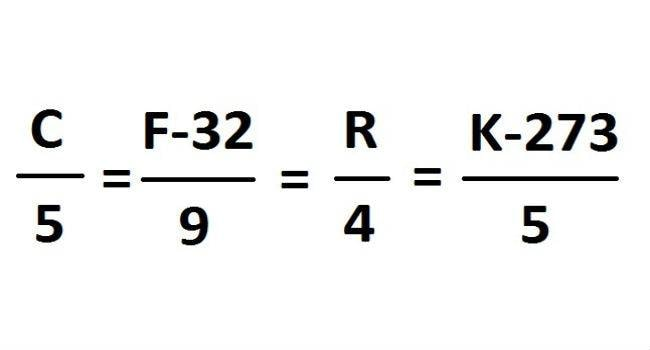# Fahrenheit to Celsius Conversion Chart

1°F = -17.22°C
(32°F − 32) × 5/9 = 0°C

## Fahrenheit

Definition: Fahrenheit (°F) is a unit of temperature that was widely used before metrication. Two points describe it: water freezes at 32°F, and water boiling point is 212°F at sea level and normal atmospheric pressure. The freezing and boiling point interval is divided into 180 parts.

## Celsius

Definition: The Celsius (°C) is an SI (International System of Units) derived temperature unit. Also called centigrade, the scale is based on 0°  for the freezing point and 100° for the boiling point of water at standard atmosphere and sea level pressure. It has a 100-degree interval between the defined point.

Fahrenheit to Celsius Conversion Chart How to convert Fahrenheit to Celsius. 0 degrees Fahrenheit is equal to –17.77778 degrees Celsius: 0 °F = -17.77778 °C. The temperature is T in degrees Celsius.

## Temperature Converter FormulaC = Celsius ; F = Fahrenheit ; R = Reaumur ; K = Kelvin

## How to Convert Fahrenheit to Celsius

The simple rule to convert Fahrenheit to Celsius is just minus 32 from the Fahrenheit value and divide by 1.8 that value.

For example,

100 Fahrenheit to Celsius.
100-32 = 68/1.8 = 37.77#### What are 25 degrees Fahrenheit equal to in Celsius?

-3.89 degrees Celsius
25 degrees Fahrenheit = -3.89 degrees Celsius.#### How do you estimate Fahrenheit to Celsius?

To estimate a Celsius temperature in Fahrenheit, multiply the temperature by 2, then add 30 to that number. This will give you an approximate temperature in Fahrenheit. If you need a more precise estimate, multiply the temperature by 2, then subtract 10% and add 32 to that number.

#### F° TO C°: FAHRENHEIT TO CELSIUS CONVERSION FORMULA

To convert temperatures in degrees Fahrenheit to Celsius, subtract 32 and multiply by .5556 (or 5/9).

• Example: (50°F – 32) x .5556 = 10°C

#### What does 45 degrees Fahrenheit equal in Celsius?

Fahrenheit 45 °F – Celsius 7.22 °C

#### What is 40 degrees celsius equal to in Fahrenheit?

40 °C Celsius  – 104.0 °F Fahrenheit

#### What is the formula for changing Fahrenheit to Celcius?

Quick Celsius (°C) / Fahrenheit (°F) Conversion:
°F to °C –  Deduct 32, then multiply by 5, then divide by 9
°C to °F – Multiply by 9, then divide by 5, then add 32

### FAHRENHEIT TO CELSIUS CONVERSION CHART

Fahrenheit

Celsius

-40°F -40°C
-30°F -34°C
-20°F -29°C
-10°F -23°C
0°F -18°C
10°F -12°C
20°F -7°C
32°F 0°C
40°F 4°C
50°F 10°C
60°F 16°C
70°F 21°C
80°F 27°C
90°F 32°C
100°F 38°C

#### C° TO F°: CELSIUS TO FAHRENHEIT CONVERSION FORMULA

To convert temperatures in degrees Celsius to Fahrenheit, multiply by 1.8 (or 9/5) and add 32.

• Example: (30°C x 1.8) + 32 = 86°F

Celsius

Fahrenheit

-40°C -40°F
-30°C -22°F
-20°C -4°F
-10°C 14°F
0°C 32°F
10°C 50°F
20°C 68°F
30°C 86°F
40°C 104°F
50°C 122°F
60°C 140°F
70°C 158°F
80°C 176°F
90°C 194°F
100°C 212°F
Categories CC